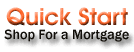Search a lender for: Select one Refinance Home Purchase Debt Consolidation Home Improvement Second Mortgage Line of Credit Home Equity Loan Construction Loan

Also known as a variable rate mortgage. The interest rate on these mortgages changes periodically.

Variable or adjustable loans are loans whose interest rate fluctuates over the period of the loan.

TERMINOLOGY
Start rate (Teaser rate):
This is the starting interest rate of the variable. It is often referred to as the teaser rate, since it is lower than the fully indexed rate. This is often done to induce people into the loan since the start rate is low.

This is the length of time for which the interest rate is fixed. Therefore if the adjustment period is six months, then the interest rate will remain fixed for six months, after which time it will adjust.

This is the maximum the interest rate can adjust up or down each adjustment period.

The maximum interest rate over the life of the loan.

Index:
This is the variable that the rate is calculated from. This is normally a number that is published in business newspapers. Well know indices include :
Prime rate: the rate offered to the bank's best customers.
Treasury bill rate: Short term debt instruments used by the U.S. Government to finance their debt. Commonly called T-bills they come in denominations of 3 months, 6 months and 1 year.
Libor: London Interbank Offered Rates. Average London Eurodollar rates.
6 month CD rate the average rate that you get when you invest in a 6 month CD.
11th District Cost of Funds: Rate determined by averaging the cost of money to banks that make the San Francisco 11th district of the Federal Reserve.

Margin:
This is a fixed number added to the index to compute the actual rate.

Conversion Options:
Some variable loans come with options to convert them to a fixed loan based on a pre-determined formula, during a given time period. For example the 1-year tbill adjustable may be converted to a fixed during the first five years on the adjustment date. The means that you could convert during the 13th, 25th, 37th, 49th and 61st months of the loan.

Computing the mortgage rate:
The formula to calculate the new interest rate is

new rate = index + margin

The new rate is also influenced by the adjustment caps and the lifetime caps.

Examples:
If the old rate was 7%, and the new rate as calculated by the formula is 9%, the actual new rate will only be 8% if the adjustment cap is 1%.
If the old rate was 7%, and the new rate as calculated by the formula is 9%, but the lifetime cap is 7.5%, then the new rate will be 7.5%.

The fully adjusted rate is equal to the index + the margin. Example if the index is 5% and the margin is 3% the fully indexed rate is 8%.

Popular Adjustable Rate Mortgage (ARM) Topics

 1 percent mortgage option arm 1 mortgage option arm interest only libor mortgage interest rate variable free download calculator mta loans good and bad Option arm mortgage Las Vegas Oregon Pay Option Arm Mortgage today's mortgage rates 5 year arm variable rate fixed payment mortgage interest tax deductable variable rate mortgage calculator What Does Variable Rate Mean what is the risk mta loanYou'll be re-directed to Top-Lenders.com
>>>>
<<<<